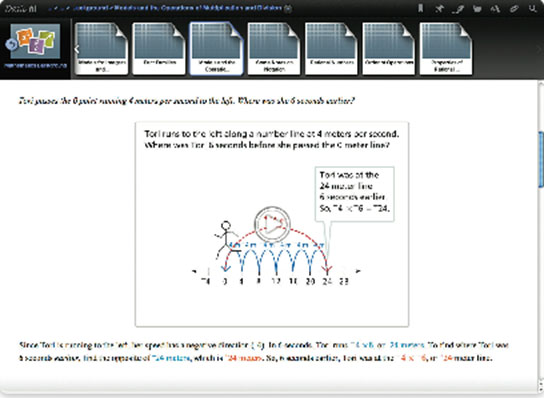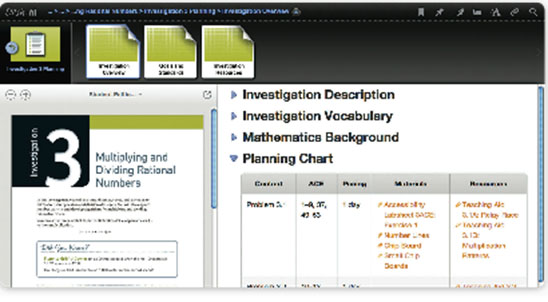Display Accessibility Tools

# Organization

These categories of support are included in the teacher support:

• Unit/Investigation/Problem descriptions
• Unit/Investigation/Problem resources
• Vocabulary lists
• Planning charts
• Goals and Standards lists
• Mathematics Background
• Launch-Explore-Summarize suggestions

## Unit Level

### Planning ChartEach Unit includes a planning chart that provides an overview of the materials, labsheets, teaching aids, assessments, and pacing for the Unit. A block pacing chart is also included.

### Goals

Two to four big concepts are identified for each Unit with an elaboration of the essential understandings for each. These goals are then listed at the beginning of each Investigation with the goals addressed in the Investigation highlighted.

### StandardsThe Common Core Content Standards addressed in the Unit are listed, followed by a list of Investigations where they are addressed. The Common Core Standards for Mathematical Practice are also listed, with a few specific examples of where they play out in the Unit. Students will be using several Mathematical Practices each day as they explore Problems.

### Mathematical BackgroundThe Mathematics Background is an overview and elaboration of the mathematics of the Unit. It includes examples and a rationale for the models and procedures used in the Unit. This background helps a teacher stand above the Unit and see the mathematics from a perspective that includes the mathematics within the particular Unit, mathematical connections to earlier Units, mathematical connections to subsequent Units and courses.

In Teacher Place, the mathematics background is enhanced with videos, animations, and series of images to give teachers a more visual exposure to the mathematics involved.

## Investigation Level

Each investigation includes a Planning Chart, Goals, Standards and Correlation of Mathematical Reflections and Goals.

### Planning ChartEach Investigation includes a planning chart similar to the Unit planning chart. In addition to providing a list of Materials and Resources, the Investigation planning chart also includes the ACE homework assignments recommended at the conclusion of each Problem.

### Goals and Standards (Investigation Level)

At the Investigation level, in addition to specifying what goals and standards from the full list of Unit goals are addressed in the Investigation, there is a correlation of the Investigation goals to the Mathematical Reflections questions for that Investigation.

### Mathematical Reflections and Goals

Each investigation ends in a Mathematical Reflections that helps students reflect on what they have learned in the investigation and how it is connected to prior understandings. As a guide for the teacher, the goals for the investigation are aligned with a corresponding question in the Mathematical Reflections. The following is an example for Investigation 3, Factorizations: Searching for Factor Strings from Prime Time.

## Mathematical Reflections

Look for evidence of student understanding of the goals for this Investigation in their responses to the questions in Mathematical Reflections. The goals addressed by each question are indicated below.

1. Why is it helpful to write a number as a product of primes?
2. Describe how you can find the prime factorization of a number.
Goals
• Recognize and use the fact that every whole number can be written in exactly one way as a product of prime numbers
• Use exponential notation to write repeated factors
1. When is it useful to find the least common multiple or greatest common factor of two or more numbers to solve a problem?
2. Describe a method for finding the least common multiple of two numbers.Is there another method? Explain.
3. Describe a method for finding the greatest common factor of two numbers. Is there another method? Explain.
Goals
• Differentiate between situations that call for common factors and situations that call for common multiples
• Develop strategies for finding factors and multiples
• Develop strategies for finding the least common multiple and the greatest common factor
• Relate the prime factorization of two numbers to the least common multiple and greatest common factor of the two numbers
• Solve problems involving factors and multiples

## Problem Level

The Problem Implementation description includes recommendations on how the teacher can set up the groups of students and for how the teacher can break up Problem parts. In addition, the appropriate materials for the Problem, including optional technology, are listed, as well as vocabulary.

### Focus Question (Problem Level)

Each Problem has a focus question that the teacher can use to guide his/her instructional decisions throughout his/her planning, teaching, and reflections on student understanding. While these questions are intended for the teacher, some teachers do share them with their students as questions they will be able to answer at the end of the Problems. In Teacher Place, the focus question is repeated at the top of every Launch, Explore, and Summarize section.

### Launch–Explore–Summarize

Actual classroom scenarios and examples of student thinking are included in the Launch, Explore, and Summarize sections to help stimulate teachers’ imaginations about what is possible.

Questions to ask students at all stages of the lesson are included to help teachers support student learning and support on-going formative assessment.The Launch, Explore, and Summarize are described in more detail in the Philosophy, Instructional Model section.